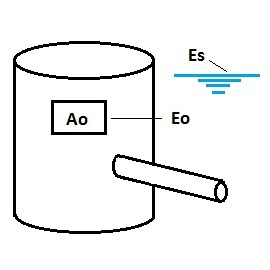* Log In to use the Calculate function * Become a Member!

Calculate Discharge from an (FHWA) Orifice Outlet Control With Free Discharge:

Calculation:
Designer/Checker:

Input:(Note: All input Metric units are converted to English units for the equations below and then the output English units are converted back to Metric)

Area of orifice (Ao - SF or m^2)
Water surface elevation at design volume (Es - ft or m)
Elevation of centroid of orifice opening (Eo - ft or m)
Orifice Coeficient (Co)

Typical values for Co: Range from 0.40 to 0.60
Rough edges - torch cut: Co = 0.40
Smooth squared edges: Co = 0.60

Output: